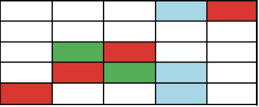# 3235. Maximal-sum Subsequence• $M_{2,1}, M_{2,2}, M_{2,3}, M_{2,4}, M_{2,5}, M_{2,6}, M_{2,7}, M_{2,8}$.
• $M_{2,2}, M_{2,3}, M_{2,4}$.
• $M_{2,6}, M_{2,7}, M_{2,8}, M_{2,1}, M_{2,2}$.
• $M_{4,3}, M_{5,3}, M_{6,3}, M_{7,3}$.
• $M_{1,2}, M_{2,3}, M_{3,4}, M_{4,5}$.
• $M_{2,4}, M_{3,3}, M_{4,2}, M_{5,1}$.
• $M_{3,3}, M_{4,2}, M_{5,1}, M_{1,5}$.
• $M_{5,6}$.

### 样例

Input
1
4
8 6 6 1
-3 4 0 5
4 2 1 9
1 -9 9 -2

Output
24


### 提示

45 人解决，68 人已尝试。

54 份提交通过，共有 267 份提交。

4.8 EMB 奖励。